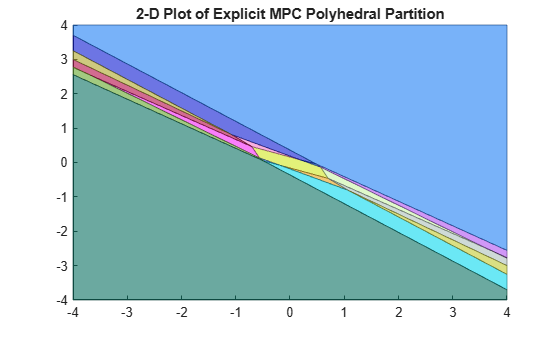# generatePlotParameters

Parameters for `plotSection`

## Syntax

``plotParams = generatePlotParameters(EMPCobj)``

## Description

example

````plotParams = generatePlotParameters(EMPCobj)` creates a structure of parameters for a 2-D sectional plot of the explicit MPC control law of the explicit MPC controller, `EMPCobj`. You set the fields of this structure and use it to generate the plot using the `plotSection` command.```

## Examples

collapse all

Define a double integrator plant model and create a traditional implicit MPC controller for this plant. Constrain the manipulated variable to have an absolute value less than `1`.

```plant = tf(1,[1 0 0]); MPCobj = mpc(plant,0.1,10,3);```
```-->The "Weights.ManipulatedVariables" property of "mpc" object is empty. Assuming default 0.00000. -->The "Weights.ManipulatedVariablesRate" property of "mpc" object is empty. Assuming default 0.10000. -->The "Weights.OutputVariables" property of "mpc" object is empty. Assuming default 1.00000. ```
`MPCobj.MV = struct('Min',-1,'Max',1);`

Define the parameter bounds for generating an explicit MPC controller.

`range = generateExplicitRange(MPCobj);`
```-->Converting the "Model.Plant" property of "mpc" object to state-space. -->Converting model to discrete time. Assuming no disturbance added to measured output channel #1. -->The "Model.Noise" property of the "mpc" object is empty. Assuming white noise on each measured output channel. ```
```range.State.Min(:) = [-10;-10]; range.State.Max(:) = [10;10]; range.Reference.Min(:) = -2; range.Reference.Max(:) = 2; range.ManipulatedVariable.Min(:) = -1.1; range.ManipulatedVariable.Max(:) = 1.1;```

Create an explicit MPC controller.

`EMPCobj = generateExplicitMPC(MPCobj,range);`
```Regions found / unexplored: 19/ 0 ```

Create a default plot parameter structure, which specifies that all of the controller parameters are fixed at their nominal values for plotting.

`plotParams = generatePlotParameters(EMPCobj);`

Allow the controller states to vary when creating a plot.

```plotParams.State.Index = []; plotParams.State.Value = [];```

Fix the manipulated variable and reference signal to `0` for plotting.

```plotParams.ManipulatedVariable.Index(1) = 1; plotParams.ManipulatedVariable.Value(1) = 0; plotParams.Reference.Index(1) = 1; plotParams.Reference.Value(1) = 0;```

Generate the 2-D section plot for the explicit MPC controller.

`plotSection(EMPCobj,plotParams)````ans = Figure (1: PiecewiseAffineSectionPlot) with properties: Number: 1 Name: 'PiecewiseAffineSectionPlot' Color: [1 1 1] Position: [348 480 583 437] Units: 'pixels' Show all properties ```

## Input Arguments

collapse all

Explicit MPC controller for which you want to create a 2-D sectional plot, specified as an Explicit MPC controller object. Use `generateExplicitMPC` to create an explicit MPC controller.

## Output Arguments

collapse all

Parameters for sectional plot of explicit MPC control law, returned as a structure.

As returned by `generatePlotParameters`, the `plotParams` structure command fixes all the control law’s parameters at their nominal values. To obtain the desired plot, eliminate the `Index` and `Value` entries of the two parameters forming the plot axes, and modify fixed values as necessary. Then, use the `plotSection` command to display the 2-D sectional plot of the explicit control law’s PWA regions with the remaining free parameters as the x and y axes.

The fields of the plot-parameters structure are as follows.

Fixed controller states, specified as a structure having an `Index` field and a `Value` field. The field `plotParams.State.Index` is a vector that contains the indices of the controller states to fix for the plot, and `plotParams.State.Value` contains the corresponding constant state values.

Modify the default value of `plotParams.State` to generate the desired plot. See Specify Fixed Parameters for 2-D Plot of Explicit Control Law.

Fixed reference signal values, specified as a structure having an `Index` field and a `Value` field. The field `plotParams.Reference.Index` is a vector that contains the indices of the reference signals to fix for the plot, and `plotParams.Reference.Value` contains the corresponding constant reference signal values.

Modify the default value of `plotParams.Reference` to generate the desired plot. See Specify Fixed Parameters for 2-D Plot of Explicit Control Law.

Fixed measured disturbance values, specified as a structure having an `Index` field and a `Value` field. The field `plotParams.MeasuredDisturbance.Index` is a vector that contains the indices of the measured disturbances to fix for the plot, and `plotParams.MeasuredDisturbance.Value` contains the corresponding constant measured disturbance values.

Modify the default value of `plotParams.MeasuredDisturbance` to generate the desired plot. See Specify Fixed Parameters for 2-D Plot of Explicit Control Law.

Fixed manipulated variable values, specified as a structure having an `Index` field and a `Value` field. The field `plotParams.ManipulatedVariable.Index` is a vector that contains the indices of the manipulated variables to fix for the plot, and `plotParams.ManipulatedVariable.Value` contains the corresponding constant manipulated variable values.

Modify the default value of `plotParams.ManipulatedVariable` to generate the desired plot. See Specify Fixed Parameters for 2-D Plot of Explicit Control Law.